Part 1 Module 1

Set Mathematics
Sets, Elements, Subsets

Any collection of objects can be considered to be a set.
We can define particular sets by listing the objects in each set. It is conventional to use set braces when doing so.

Examples of sets:

{a, b, c, d, e, f}

{4, 9, 2, 7}

{Larry, Moe, Curly}

{cat, dog}

{1, 2, 3, 4, 5, 6, 7, ...}

{the people in this room}

NAMES FOR SETS

We will typically use upper case letters for the names of sets.

Let

A = {a, b, c, d, e, f}

B = {4, 9, 2, 7}

C = {Larry, Moe, Curly}

D = {cat, dog}

N = {1, 2, 3, 4, 5, 6, 7, ...}

F = {the people in this room}

ELEMENTS OR MEMBERS OF SETS

The contents of a set are called its elements or members.

We say "9 is an element of B"

"cat is an element of D"

Notation:Likewise:

6 is not an element of B

dog is not an element of AThe CARDINALITY of a set is the number of elements in the set.

In general the cardinality of a set S is denoted n(S).

For example, the cardinality of set B is 4.

Notation:

n(B) = 4

Likewise n(A) = 6

n(D) = 2

n(F) is _____

n(N) is infinity?

SET EQUALITY

Two sets are said to be equal if they contain exactly the same elements.

Examples

{a, b, c} = {b, c, a}

{4, 2, 7, 9} = B

On the other hand:A formal definition of set equality is this:

Sets S and T are equal if every element of S is an element of T and every element of T is an element of S.

Set-builder notation
is a formalistic way of describing the elements of a set without listing them all. It is useful in some cases where a less formal description might be ambiguous.

EXAMPLE 1.1.1
F = {x|x is a person in this room}

"F is the set of all x such that x is a person in this room"

More examples of set-builder notation:

N = {x|x is a positive integer}

"N is the set of all x such that x is a positive integer"

New set:
E = {x|x is a positive even number}

List the elements of E.

SUBSETS

Let A = {a, b, c, d, e, f}
B = {4, 9, 2, 7}
C = {Larry, Moe, Curly}
D = {cat, dog}
E = {2, 4, 6, 8, 10, ...}
N = {1, 2, 3, 4, 5, 6, 7, ...}
F = {x|x is a person in this room}
G = {b, c, f}

Notice, for instance, that every element of G is also an element of A.

In a case like this we say "G is a subset of A"

Notation:Likewise,A formal definition of the word subset is this:

For sets S and T, S is a subset of T if every element of S is also an element of T.
This means that S is contained within T.

ExampleAlso:Formally: S is not a subset of T if there is at least one element of S that is not an element of T.

EXAMPLE 1.1.2

Referring to the sets listed earlier, determine whether each statement is true or false.

1.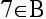2. n(A) = a

3.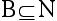4.5.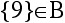6.7.See solutions

Exercise #7 above illustrates a general fact about subsets:

Every set is a subset of itself.

PROPER SUBSETS

If S is a subset of T but is not equal to T, we say that S is a proper subset of T.

Notation:Examples: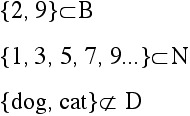The last example illustrates this basic fact:

No set is a proper subset of itself.

COMPLEMENTS, THE UNIVERSAL SET, AND THE EMPTY SET

Consider the set S = {Moe, Larry}

Suppose we want to complete the following exercise:

List all of the elements that are NOT in S.

Are we supposed to list...

...the names of all film performers except Moe and Larry?

...the names of all stooges except Moe and Larry?

...every object on Earth except Moe and Larry?

Unless we establish some boundaries on the scope of this exercise, we cannot finish it.

To establish a frame of reference for a set problem, we can define a universal set (U) for the problem:

For any set problem or discussion, a universal set (U) is a "larger" set that contains all of the elements that may be of interest in the discussion; in particular, the universal set at least contains all of the elements of all of the sets in the discussion.

Returning to the example at hand:

Let U = {Moe, Larry, Curly, Shemp, Curly Joe}

Then to list the elements that are not in S, we list those elements of U that are not in S:

{Curly, Shemp, Curly Joe}

This set is called the complement of S, and is denoted.

THE EMPTY SET

There is a unique set that contains no elements. It is called the empty set or the null set or the void set.

The empty set is usually denoted with one of these symbols:

{ } or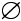EXAMPLE 1.1.3

Let U = {1, 2, 3, 4, 5, 6, 7, 8, 9, 10}

X = {2, 4, 6, 8, 10}

W = {x|x is an odd number}

Y = {3}

True or false: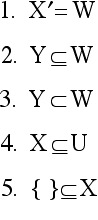see solution

GENERAL FACT:

In any set problem, every set is a subset of U, and { } is a subset of every set.

WWW Note:
For practice on problems involving sets, elements, subsets and the empty set, visit The Sets Appealer

THE NUMBER OF SUBSETS IN A FINITE SET

General observation: It makes sense to assume that the more elements a set has, the more subsets it will have.

For finite sets, there is a strict relationship between the cardinality of a set and the number of subsets . We can discover this relationship by filling in the following table: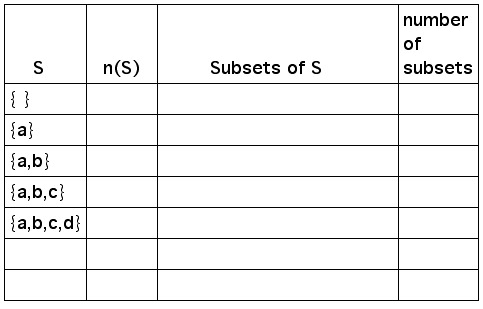see the completed table

The number of subsets in a finite set

If n(S) = k, then the number of subsets in S is 2k.

EXAMPLE 1.1.4

A = {a, b, c, d, e, f}

Since n(A) = 6, A has 26 subsets. That is, A has 64 subsets (26 = 64).

How many proper subsets does A have?

EXAMPLE 1.1.5

Let U = {1, 2, 3, 4, 5, 6, 7,...}

Let S = {x|x is less than 10}

1. How many subsets does S have?

2. How many proper subsets does S have?

see solution

WWW note:

For exercises involving the number of subsets and the number of proper subsets, try The Subsetizer.